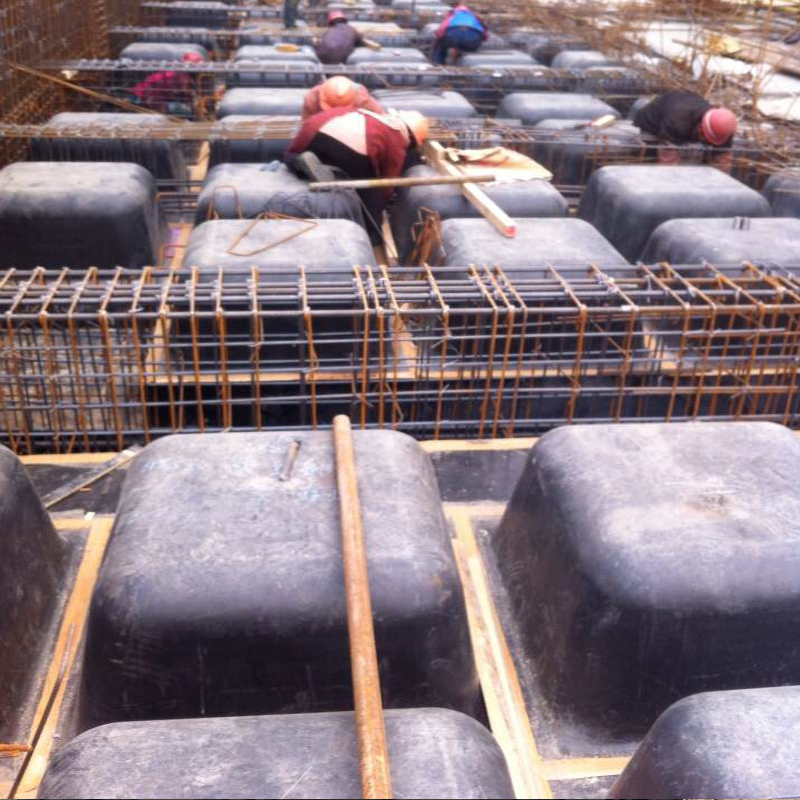一次性建筑模壳

以东平县老湖镇塑胶厂某地下室工程为例，一次性建筑模壳施工搭设满堂脚手架的操作要点：

本工程为3#楼南地下室及商业网点，高层4.9m，大跨度10.1m。根据实际情况，脚手架平面布置纵横间距700~800，若遇梁，则在梁两侧；立面布置，地面以上200道，以上每1800一道；设剪刀撑，须由底到顶连续设置，跨度大于6m的须在跨中成十字型设竖向剪刀撑。旋转扣件和对接扣件的抗滑必须确保拧紧牢固，必要时增设构件加固。4m立杆采用32长60cm顶丝，4.2m立杆采用28长50cm顶丝。通过计算，满足荷载要求。参数信息:

1.脚手架参数

横向间距或排距(m):0.80；纵距(m):0.80；步距(m):1.80；

立杆上端伸出至模板支撑点长度(m):0.40；脚手架搭设高度(m):4.0~4.2；

采用的钢管(mm):Φ48×3.0 ；

扣件连接方式:双扣件，扣件抗滑承载力系数:0.80；

板底支撑连接方式:方木支撑；

2.荷载参数

模板与木板自重(kN/m2):0.350；混凝土与钢筋自重(kN/m3):25.000；

楼板浇筑厚度(m):0.400或0.620；倾倒混凝土荷载标准值(kN/m2):2.000；

施工均布荷载标准值(kN/m2):1.000；

3.木方参数

木方弹性模量E(N/mm2):9500.000；木方抗弯强度设计值(N/mm2):13.000；

木方抗剪强度设计值(N/mm2):1.300；木方的间隔距离(mm):100.000；

木方的截面宽度(mm):50.00；木方的截面高度(mm):100.00；

模板支撑方木的计算:

方木按照简支梁计算，方木的截面力学参数为

本算例中，方木的截面惯性矩I和截面抵抗矩W分别为:

W=5.000×10.000×10.000/6 = 83.33 cm3；

I=5.000×10.000×10.000×10.000/12 = 416.67 cm4；

1.荷载的计算：

(1)百替模壳楼盖按钢筋混凝土板自重(kN/m)：

q1= 25.000×0.400×0.100 = 1.000 kN/m；

(2)模板的自重荷载(kN/m):

q2= 0.350×0.100 = 0.035 kN/m ；

(3)活荷载为施工荷载标准值与振倒混凝土时产生的荷载(kN)：

p1 = (1.000+2.000)×0.800×0.100 = 0.240 kN；

2.强度计算:

大弯矩考虑为静荷载与活荷载的计算值不利分配的弯矩和，计算公式如下:

均布荷载   q =  1.2×(1.000 + 0.035) = 1.242 kN/m；

集中荷载   p = 1.4×0.240=0.036 kN；

大弯距   M = Pl/4 + ql2/8 = 1.134×0.800 /4 + 1.242×0.8002/8 = 0.326 kN.m；

大支座力 N = P/2 + ql/2 = 1.134/2 + 1.242×0.800/2 = 1.064 kN ；

截面应力   σ= M / w = 0.541×106/83.333×103 = 6.495 N/mm2；

方木的计算强度为 6.495 小13.0 N/mm2,满足要求!

3.抗剪计算：

大剪力的计算公式如下:Q = ql/2 + P/2

截面抗剪强度必须满足:T = 3Q/2bh < [T]

其中大剪力: Q = 0.800×1.242/2+1.134/2 = 1.064kN；

截面抗剪强度计算值 T = 3 ×1063.8/(2 ×50.000 ×100.000) = 0.319N/mm2；

截面抗剪强度设计值 [T] = 1.300 N/mm2；

方木的抗剪强度为0.319小于 1.300 ，满足要求!

4.挠度计算:

大弯矩考虑为静荷载与活荷载的计算值不利分配的挠度和，计算公式如下:

均布荷载   q = q1 + q2 = 2.250+0.105=2.355 kN/m；

集中荷载   p = 0.810 kN；

大变形   V= 5×2.355×900.0004 /(384×9500.000×4166666.67) +

810.000×900.0003 /( 48×9500.000×4166666.67)  = 0.819 mm；

方木的大挠度 0.819 小于 900.000/250,满足要求!

木方支撑钢管计算:

支撑钢管按照集中荷载作用下的三跨连续梁计算；

集中荷载P取纵向板底支撑传递力，P = 2.826×0.900 + 1.134 = 3.677 kN；

大弯矩 Mmax = 0.883 kN.m ；

大变形 Vmax = 2.042 mm ；

大支座力 Qmax = 12.013 kN ；

截面应力 σ= 0.883×106/5080.000=173.779 N/mm2 ；

支撑钢管的计算强度小于 205.000 N/mm2,满足要求!

支撑钢管的大挠度小于900.000/150与10 mm,满足要求!

扣件抗滑移的计算:

按照《建筑施工扣件式钢管脚手架安全技术规范培训讲座》刘群主编，P96页，双扣件承载力设计值取16.00kN，

按照扣件抗滑承载力系数0.80，该工程实际的旋转双扣件承载力取值为12.80kN 。

纵向或横向水平杆传给立杆的竖向作用力设计值 R= 12.013 kN；

R < 12.80 kN,所以双扣件抗滑承载力的设计计算满足要求!

模板支架荷载标准值(轴力):

作用于模板支架的荷载包括静荷载、活荷载和风荷载。

1.静荷载标准值包括以下内容：

(1)脚手架的自重(kN)：NG1 = 0.129×4.350 = 0.562 kN；

(2)模板的自重(kN)：NG2 = 0.350×0.800×0.800 = 0.224 kN；

(3)钢筋混凝土楼板自重(kN)：NG3 = 25.000×0.400×0.800×0.800 = 6.400 kN；

经计算得到，静荷载标准值 NG = NG1+NG2+NG3 = 7.186 kN；

2.活荷载为施工荷载标准值与振倒混凝土时产生的荷载。经计算得到，活荷载标准值 NQ = (1.000+2.000 ) ×0.800×0.800 = 1.920 kN；

3.立杆的轴向压力设计值计算公式 N = 1.2NG + 1.4NQ = 11.311 kN；

立杆的稳定性计算:

立杆的稳定性计算公式:

其中  N ---- 立杆的轴心压力设计值(kN) ：N = 11.311 kN；

σ---- 轴心受压立杆的稳定系数,由长细比 lo/i 查表得到；

i ---- 计算立杆的截面回转半径(cm) ：i = 1.58 cm；

A ---- 立杆净截面面积(cm2)：A = 4.89 cm2；

W ---- 立杆净截面模量(抵抗矩)(cm3)：W=5.08 cm3；

σ-------- 钢管立杆抗压强度计算值 (N/mm2)；

[f]---- 钢管立杆抗压强度设计值 ：[f] =205.000 N/mm2；

Lo---- 计算长度 (m)；

如果完全参照《扣件式规范》，由公式(1)或(2)计算

lo = k1uh                    (1)

lo = (h+2a)                  (2)

k1---- 计算长度附加系数，取值为1.155；

u ---- 计算长度系数，参照《扣件式规范》表5.3.3；u = 1.700；

a ---- 立杆上端伸出顶层横杆中心线至模板支撑点的长度；a = 0.100 m；

公式(1)的计算结果：

立杆计算长度 Lo = k1uh = 1.155×1.700×1.500 = 2.945 m；

Lo/i = 2945.250 / 15.800 = 186.000 ；

由长细比 Lo/i  的结果查表得到轴心受压立杆的稳定系数φ= 0.207 ；

钢管立杆受压强度计算值 ；σ=11706.102/（0.207×489.000） = 115.647 N/mm2；

立杆稳定性计算 σ= 115.647 N/mm2 小于 [f] = 205.000满足要求！

公式(2)的计算结果：

立杆计算长度 Lo = h+2a = 1.500+0.100×2 = 1.700 m；

Lo/i = 1700.000 / 15.800 = 108.000 ；

由长细比 Lo/i  的结果查表得到轴心受压立杆的稳定系数φ= 0.530 ；

钢管立杆受压强度计算值 ；σ=11706.102/（0.530×489.000） = 45.168 N/mm2；

立杆稳定性计算 σ= 45.168 N/mm2 小于 [f] = 205.000满足要求！

如果考虑到高支撑架的安全因素，适宜由公式(3)计算

lo = k1k2(h+2a)              (3)

k1 -- 计算长度附加系数按照表1取值1.243；

k2 -- 计算长度附加系数，h+2a = 1.700 按照表2取值1.001 ；

公式(3)的计算结果：

立杆计算长度 Lo = k1k2(h+2a) = 1.243×1.001×(1.500+0.100×2) = 2.115 m；

Lo/i = 2115.213 / 15.800 = 134.000 ；

由长细比 Lo/i  的结果查表得到轴心受压立杆的稳定系数φ= 0.376 ；

钢管立杆受压强度计算值 ；σ=11706.102/（0.376×489.000） = 63.667 N/mm2；

立杆稳定性计算 σ= 63.667 N/mm2 小于 [f] = 205.000满足要求！